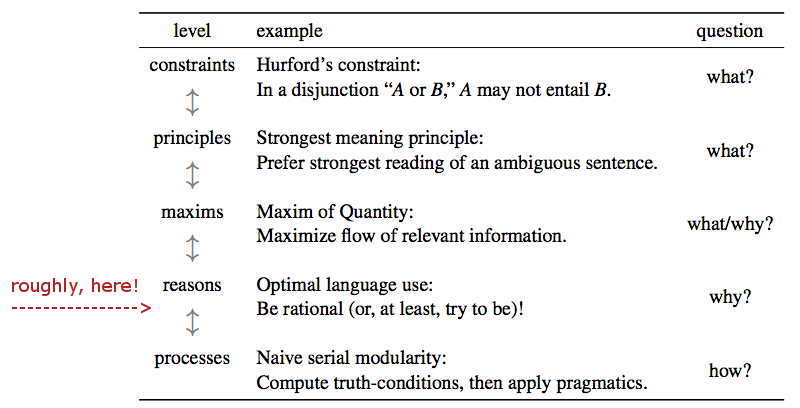$\definecolor{firebrick}{RGB}{178,34,34} \newcommand{\red}{{\color{firebrick}{#1}}}$ $\definecolor{green}{RGB}{107,142,35} \newcommand{\green}{{\color{green}{#1}}}$ $\definecolor{blue}{RGB}{0,0,205} \newcommand{\blue}{{\color{blue}{#1}}}$ $\newcommand{\den}{[\![#1]\!]}$ $\newcommand{\set}{\{#1\}}$

## overview

• what is probabilistic pragmatics?

• example: vanilla rational speech act model

• extensions & applications:
• individual differences
• embedded scalars

## what is probabilistic pragmatics?

• definitely one thing: a piece of bad terminology
• probabilities play a role, but they are not the main thing

• blunt umbrella term for a set of approaches unified by family resemblance
• game theoretic pragmatics, "Bayesian pragmatics"
• modern post-Grice (Geurts, Lauer, Optimality or Relevance Theory)

• gradient concept characterized by a property cluster

Franke & Jäger (2016), Probabilistic pragmatics, ZfS 35(1):3-44

## key properties

1. probabilistic
• language users are usually uncertain about relevant contextual elements
• probabilities are a good tool to capture uncertainty
2. interactive
• explicitly consider speaker and listener perspectives
3. rationalistic
• language use as goal-oriented, purposeful behavior
4. computational
• formally precise, implementable (likely: quantitative) formulations
5. data-oriented
• pragmatic theory feeds full data-generating model for experimental data

$$\Rightarrow$$ Bayesian as a consequence of particular implementations of 1-3

## levels of analysis## rational analysis

A rational analysis is an explanation of an aspect of human behavior based
on the assumption that it is optimized somehow to the structure of the
environment. ... [T]he term does not imply any actual logical deduction in
choosing optimal behavior, only that the behavior will be optimized.
(Anderson 1991, p. 471)

• speaker and listener observe a fixed set $$T$$ of referents, e.g.:• speaker knows which referent $$t \in T$$ she wants to talk about

• speaker can choose a message $$m$$ from set $$M = \{ \text{blue}, \text{green}, \text{square}, \text{circle} \}$$

• listener tries to recover intended referent based on message

• communication is successful if guess matches intended referent

[signaling game!]

## rational speech act model

dummy

literal listener picks literal interpretation (uniformly at random):

$P_{LL}(t \mid m) \propto P(t \mid [\![m]\!])$

dummy

Gricean speaker approximates informativity-maximization (with parameter $$\lambda$$):

$P_{S}(m \mid t \, ; \, \lambda) \propto \exp(\lambda \cdot \log P_{LL}(t \mid m))$

dummy

pragmatic listener uses Bayes' rule to infer likely world states:

$P_L(t \mid m \, ; \, \lambda) \propto P(t) \cdot P_S(m \mid t \, ; \, \lambda)$

(c.f., Benz 2006, Frank & Goodman 2012)